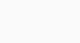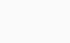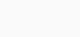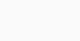# 1. Linear Equations in Two Variables

## Practice Set 1.2

1.Linear Equations in Two Variables

#### 1. Complete the following table to draw graph of the equations

##### (II) x – y = 4

Solution:

(x+y=3)(x, y) = (3, 0) and x = 3                  ……..(Given)

∴y = 0

Substituting

y = 5

x + 5 = 3

∴x = – 2

(x, y) = (0, 3) and y = 3 (Given)

∴x = 0

(x-y=4)Substituting y = 0

x – 0 = 4

∴x = 4

Substituting x = -1

– 1 – y = 4

∴y = – 5

#### 2. Solve the following simultaneous equations graphically.

##### (1) x + y = 6 ; x – y = 4

Solution:

x+y=6x-y=4##### (2) x + y = 5 ; x – y = 3

Solution:

x+y=5 ∴∴y=5-xx-y=3∴ y=x-3x-3The coordinates of the point of intersection are (4, 1).

Ans. The solution of the given simultaneous equations is x = 4 , y = 1 .

##### (3) x + y = 0 ; 2x – y = 9

Solution:

x + y = 0

∴y = – x2x – y = 9

∴y = 2x – 9The coordinates of the point of intersection are (3, -3)

Ans. The solution of the given simultaneous equations is x = 3 , y = -3 .

##### (4) 3x – y = 2 ; 2x – y = 3

Solution:

3x – y = 2

∴3x – 2 = y

∴y = 3x – 22x – y = 3

∴y = 2x – 3The coordinates of the point of intersection

are (-1,-5).

Ans. The solution of the given simultaneous equations is x = – 1, y = – 5 .

##### (5) 3x – 4y = -7 ; 5x – 2y = 0

Solution:

3x – 4y = – 7

∴3x + 7 = 4y5x – 2y = 0

∴5x = 2yThe coordinates of the point of intersection

are (1, 2.5).

Ans. The solution of the given simultaneous equations is x = 1, y = 2.5 .

##### (6) 2x – 3y = 4 ; 3y – x = 4

Solution:

2x – 3y = 4

∴2x – 4 = 3y3y-x-4

∴3y = x + 4The coordinates of the point of intersection are (8, 4).

Ans. The solution of the given simultaneous equations is x = 8 , y = 4

1.Linear Equations in Two Variables